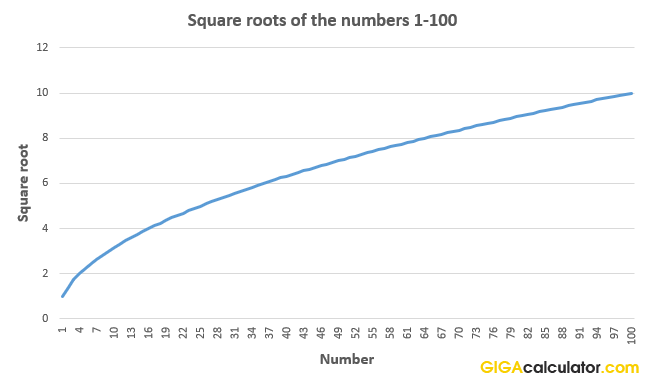# Square Root Calculator

Use this online calculator to easily calculate the square root of a given number, including fractions. Quick and easy square root finder.

Share calculator:

Embed this tool:
get code

## What is a square root?

The square root of a number answers the question "what number can I multiply by itself to get this number?". It is the reverse of the exponentiation operation with an exponent of 2, so if r2 = x, then we say that "r is the root of x". Finding the root of a number has a special notation called the radical symbol: √. Usually the radical spans over the entire equation for which the root is to be found. It is called a "square" root since multiplying a number by itself is called "squaring" as it is how one finds the area of a square.

For every positive number there are two square roots - one positive and one negative. For example, the square root of 4 is 2, but also -2, since -2 x -2 = 4. The negative root is always equal in value to the positive one, but opposite in sign. You can see examples in the table of common roots below. Most often when talking about "the root of" some number, people refer to the Principal Square Root which is always the positive root. This is the number our square root calculator outputs as well.

In geometrical terms, the square root function maps the area of a square onto its side length. The function √x is continuous for all nonnegative x and differentiable for all positive x.

## How calculate a square root

Unlike other mathematical tasks, there is no formula or single best way to calculate the square root of a real number. Still, there is a handy way to find the square root of a number manually. The algorithm to find √a is as follows:

1. Start with a guess (b). If a is between two perfect squares, a good guess would be a number between those squares.
2. Divide a by b: e = a / b. If the estimate e is an integer, stop. Also stop if the estimate has achieved the desired level of decimal precision.
3. Get a new guess b1 by averaging the result of step #2 e and the initial guess b: b1 = (e + b) / 2
4. Go to step #2 using b1 in place of b

For example, to find the square root of 30 with a precision of three numbers after the decimal point:

Step 1: a = 30 is between 25 and 36, which have roots of 5 and 6 respectively. Let us start with b = 5.5.
Step 2: e = a / b = 30 / 5.5 = 5.45(45). Since b is not equal to e (5.500 ≠ 5.454), continue calculation.
Step 3: b1 = (5.45 + 5.5) / 2 = 5.47727(27)
Step 4: e = 30 / 5.47727 = 5.477178. Since b1 = e = 5.477 within three position after the decimal point, stop the square root-finding algorithm with a result of √30 = 5.47727(27).

Checking the outcome against the square root calculator output of 5.477226 reveals that the algorithm resulted in a correct solution. While the above process can be fairly tedious especially with larger roots, but will help you find the square root of any number with the desired decimal precision.

## Properties and practical application of square roots

Square roots appear frequently in mathematics, geometry and physics. For example, many physical forces measured in quantities or intensities diminish inversely proportional to the square root of the distance. So, gravity between two objects 4 meters apart will be 1/√4 relative to their gravity at 0 meters. The same is true for radar energy waves, radio waves, light and magnetic radiation in general, and sound waves in gases. It is usually referred to as the "inverse-square law".

The square root is key in probability theory and statistics where it defines the fundamental concept of standard deviation.Plotting the results from the square root function, as calculated using this square root calculator, on a graph reveals that it has the shape of half a parabola.

## Commonly used square roots

Table of commonly encountered square roots:

Commonly used square roots
Square Root ofPositive rootNegative root
√1 1 -1
√2 1.4142 -1.4142
√3 1.7321 -1.7321
√4 2 -2
√5 2.2361 -2.2361
√8 2.8284 -2.8284
√9 3 -3
√10 3.1623 -3.1623
√16 4 -4
√25 5 -5
√36 6 -6
√49 7 -7
√64 8 -8
√81 9 -9
√100 10 -10
√1024 32 -32

The calculations were performed using this calculator.

## Does the calculator support fractions?

Yes, simply enter the fraction as a decimal number (use dot as a separator) and you will get the corresponding root. For example, to compute the square root of 1/4 simply enter 0.25 in the number field, press "Calculate" and you will get 0.50 as ouput. If you are having difficulty converting a fraction to a decimal number, you will find our fraction to decimal converter handy.

#### Cite this calculator & page

If you'd like to cite this online calculator resource and information as provided on the page, you can use the following citation:
Georgiev G.Z., "Square Root Calculator", [online] Available at: https://www.gigacalculator.com/calculators/square-root-calculator.php URL [Accessed Date: 01 Apr, 2023].